# Mean State

Period Mean (original grids) [W/m2]
Model Period Mean (intersection) [W/m2]
Model Period Mean (complement) [W/m2]
Benchmark Period Mean (intersection) [W/m2]
Benchmark Period Mean (complement) [W/m2]
Bias [W/m2]
RMSE [W/m2]
Phase Shift [months]
Bias Score 
RMSE Score 
Seasonal Cycle Score 
Spatial Distribution Score 
Interannual Variability Score 
Overall Score 
Benchmark [-] 131.
CLM4 [-] 141. 141. 130. 157. 11.5 24.1 1.33 0.395 0.449 0.828 0.920 0.548 0.598
CLM4.5 [-] 137. 137. 130. 157. 7.82 23.0 1.32 0.435 0.441 0.828 0.956 0.556 0.610
CLM5 [-] 136. 136. 130. 157. 6.69 21.4 1.30 0.462 0.452 0.832 0.980 0.529 0.618
Period Mean (original grids) [W/m2]
Model Period Mean (intersection) [W/m2]
Model Period Mean (complement) [W/m2]
Benchmark Period Mean (intersection) [W/m2]
Benchmark Period Mean (complement) [W/m2]
Bias [W/m2]
RMSE [W/m2]
Phase Shift [months]
Bias Score 
RMSE Score 
Seasonal Cycle Score 
Spatial Distribution Score 
Interannual Variability Score 
Overall Score 
Benchmark [-] 123.
CLM4 [-] 114. 113. 122. 160. -8.38 24.5 1.54 0.589 0.458 0.765 0.958 0.669 0.649
CLM4.5 [-] 110. 109. 122. 160. -12.2 25.2 1.56 0.562 0.458 0.763 0.996 0.675 0.652
CLM5 [-] 109. 108. 122. 160. -13.3 25.3 1.52 0.550 0.470 0.767 0.995 0.625 0.646
Period Mean (original grids) [W/m2]
Model Period Mean (intersection) [W/m2]
Model Period Mean (complement) [W/m2]
Benchmark Period Mean (intersection) [W/m2]
Benchmark Period Mean (complement) [W/m2]
Bias [W/m2]
RMSE [W/m2]
Phase Shift [months]
Bias Score 
RMSE Score 
Seasonal Cycle Score 
Spatial Distribution Score 
Interannual Variability Score 
Overall Score 
Benchmark [-] 123.
CLM4 [-] 118. 118. 121. 157. -2.25 19.6 1.22 0.712 0.627 0.838 0.996 0.603 0.734
CLM4.5 [-] 113. 113. 121. 157. -6.80 20.5 1.18 0.685 0.623 0.848 0.993 0.619 0.732
CLM5 [-] 114. 114. 121. 157. -5.34 20.3 1.24 0.703 0.612 0.836 0.995 0.569 0.721
Period Mean (original grids) [W/m2]
Model Period Mean (intersection) [W/m2]
Model Period Mean (complement) [W/m2]
Benchmark Period Mean (intersection) [W/m2]
Benchmark Period Mean (complement) [W/m2]
Bias [W/m2]
RMSE [W/m2]
Phase Shift [months]
Bias Score 
RMSE Score 
Seasonal Cycle Score 
Spatial Distribution Score 
Interannual Variability Score 
Overall Score 
Benchmark [-] 34.4
CLM4 [-] 14.6 14.7 34.5 33.0 -20.5 27.9 0.588 0.697 0.721 0.961 0.906 0.681 0.781
CLM4.5 [-] 18.8 18.8 34.5 33.0 -17.1 25.1 0.468 0.743 0.733 0.969 0.806 0.659 0.774
CLM5 [-] 15.2 15.2 34.5 33.0 -20.7 27.5 0.421 0.699 0.729 0.972 0.779 0.657 0.761
Period Mean (original grids) [W/m2]
Model Period Mean (intersection) [W/m2]
Model Period Mean (complement) [W/m2]
Benchmark Period Mean (intersection) [W/m2]
Benchmark Period Mean (complement) [W/m2]
Bias [W/m2]
RMSE [W/m2]
Phase Shift [months]
Bias Score 
RMSE Score 
Seasonal Cycle Score 
Spatial Distribution Score 
Interannual Variability Score 
Overall Score 
Benchmark [-] 147.
CLM4 [-] 164. 164. 145. 156. 21.3 28.1 1.53 0.334 0.438 0.773 0.998 0.524 0.584
CLM4.5 [-] 162. 162. 145. 156. 20.2 27.2 1.56 0.350 0.439 0.767 0.996 0.518 0.585
CLM5 [-] 157. 157. 145. 156. 14.9 23.6 1.65 0.418 0.438 0.755 0.998 0.507 0.592
Period Mean (original grids) [W/m2]
Model Period Mean (intersection) [W/m2]
Model Period Mean (complement) [W/m2]
Benchmark Period Mean (intersection) [W/m2]
Benchmark Period Mean (complement) [W/m2]
Bias [W/m2]
RMSE [W/m2]
Phase Shift [months]
Bias Score 
RMSE Score 
Seasonal Cycle Score 
Spatial Distribution Score 
Interannual Variability Score 
Overall Score 
Benchmark [-] 53.7
CLM4 [-] 49.1 49.1 53.5 64.9 -4.36 19.4 0.379 0.873 0.747 0.975 0.742 0.772 0.809
CLM4.5 [-] 46.8 46.8 53.5 64.9 -6.64 21.3 0.367 0.850 0.733 0.976 0.681 0.776 0.792
CLM5 [-] 43.0 42.9 53.5 64.9 -10.5 22.6 0.391 0.832 0.719 0.974 0.861 0.783 0.814
Period Mean (original grids) [W/m2]
Model Period Mean (intersection) [W/m2]
Model Period Mean (complement) [W/m2]
Benchmark Period Mean (intersection) [W/m2]
Benchmark Period Mean (complement) [W/m2]
Bias [W/m2]
RMSE [W/m2]
Phase Shift [months]
Bias Score 
RMSE Score 
Seasonal Cycle Score 
Spatial Distribution Score 
Interannual Variability Score 
Overall Score 
Benchmark [-] 71.7
CLM4 [-] 70.3 70.1 71.1 90.6 0.201 21.3 0.515 0.775 0.707 0.957 0.875 0.715 0.789
CLM4.5 [-] 68.8 68.6 71.1 90.6 -1.17 21.6 0.488 0.769 0.706 0.959 0.883 0.714 0.790
CLM5 [-] 66.2 66.0 71.1 90.6 -3.81 21.4 0.517 0.789 0.693 0.955 0.944 0.733 0.801
Period Mean (original grids) [W/m2]
Model Period Mean (intersection) [W/m2]
Model Period Mean (complement) [W/m2]
Benchmark Period Mean (intersection) [W/m2]
Benchmark Period Mean (complement) [W/m2]
Bias [W/m2]
RMSE [W/m2]
Phase Shift [months]
Bias Score 
RMSE Score 
Seasonal Cycle Score 
Spatial Distribution Score 
Interannual Variability Score 
Overall Score 
Benchmark [-] 120.
CLM4 [-] 126. 126. 118. 153. 11.9 25.9 0.930 0.576 0.556 0.884 0.934 0.689 0.699
CLM4.5 [-] 122. 122. 118. 153. 8.84 24.3 0.928 0.632 0.556 0.884 0.939 0.672 0.707
CLM5 [-] 121. 121. 118. 153. 7.47 23.1 0.885 0.646 0.568 0.896 0.958 0.615 0.709
Period Mean (original grids) [W/m2]
Model Period Mean (intersection) [W/m2]
Model Period Mean (complement) [W/m2]
Benchmark Period Mean (intersection) [W/m2]
Benchmark Period Mean (complement) [W/m2]
Bias [W/m2]
RMSE [W/m2]
Phase Shift [months]
Bias Score 
RMSE Score 
Seasonal Cycle Score 
Spatial Distribution Score 
Interannual Variability Score 
Overall Score 
Benchmark [-] 30.5
CLM4 [-] 11.6 12.0 31.0 19.5 -20.0 26.1 0.442 0.695 0.735 0.971 0.911 0.680 0.788
CLM4.5 [-] 13.1 13.5 31.0 19.5 -18.7 26.2 0.459 0.714 0.717 0.970 0.872 0.700 0.782
CLM5 [-] 10.3 10.7 31.0 19.5 -21.6 27.9 0.400 0.676 0.724 0.973 0.853 0.708 0.777
Period Mean (original grids) [W/m2]
Model Period Mean (intersection) [W/m2]
Model Period Mean (complement) [W/m2]
Benchmark Period Mean (intersection) [W/m2]
Benchmark Period Mean (complement) [W/m2]
Bias [W/m2]
RMSE [W/m2]
Phase Shift [months]
Bias Score 
RMSE Score 
Seasonal Cycle Score 
Spatial Distribution Score 
Interannual Variability Score 
Overall Score 
Benchmark [-] 109.
CLM4 [-] 100. 99.9 108. 131. -6.28 16.9 0.715 0.827 0.735 0.938 0.979 0.666 0.813
CLM4.5 [-] 99.7 99.7 108. 131. -6.52 17.2 0.749 0.822 0.731 0.933 0.970 0.679 0.811
CLM5 [-] 98.8 98.7 108. 131. -7.39 17.6 0.707 0.816 0.730 0.941 0.985 0.683 0.814
Period Mean (original grids) [W/m2]
Model Period Mean (intersection) [W/m2]
Model Period Mean (complement) [W/m2]
Benchmark Period Mean (intersection) [W/m2]
Benchmark Period Mean (complement) [W/m2]
Bias [W/m2]
RMSE [W/m2]
Phase Shift [months]
Bias Score 
RMSE Score 
Seasonal Cycle Score 
Spatial Distribution Score 
Interannual Variability Score 
Overall Score 
Benchmark [-] 65.7
CLM4 [-] 65.3 65.3 63.8 85.4 2.11 21.9 0.360 0.829 0.733 0.976 0.934 0.802 0.834
CLM4.5 [-] 66.7 66.8 63.8 85.4 3.45 22.0 0.321 0.838 0.725 0.979 0.965 0.799 0.838
CLM5 [-] 62.1 62.2 63.8 85.4 -0.888 22.1 0.271 0.856 0.716 0.982 0.960 0.804 0.839
Period Mean (original grids) [W/m2]
Model Period Mean (intersection) [W/m2]
Model Period Mean (complement) [W/m2]
Benchmark Period Mean (intersection) [W/m2]
Benchmark Period Mean (complement) [W/m2]
Bias [W/m2]
RMSE [W/m2]
Phase Shift [months]
Bias Score 
RMSE Score 
Seasonal Cycle Score 
Spatial Distribution Score 
Interannual Variability Score 
Overall Score 
Benchmark [-] 109.
CLM4 [-] 75.1 75.1 80.5 123. -3.52 22.4 0.729 0.665 0.623 0.918 0.963 0.625 0.736
CLM4.5 [-] 74.2 74.2 80.5 123. -4.25 22.2 0.726 0.675 0.620 0.918 0.971 0.626 0.738
CLM5 [-] 72.0 72.0 80.5 123. -6.85 22.6 0.734 0.663 0.620 0.917 0.963 0.618 0.734
Period Mean (original grids) [W/m2]
Model Period Mean (intersection) [W/m2]
Model Period Mean (complement) [W/m2]
Benchmark Period Mean (intersection) [W/m2]
Benchmark Period Mean (complement) [W/m2]
Bias [W/m2]
RMSE [W/m2]
Phase Shift [months]
Bias Score 
RMSE Score 
Seasonal Cycle Score 
Spatial Distribution Score 
Interannual Variability Score 
Overall Score 
Benchmark [-] 76.6
CLM4 [-] 64.2 64.2 73.9 144. -7.62 19.8 0.913 0.680 0.622 0.899 0.937 0.599 0.726
CLM4.5 [-] 64.8 64.8 73.9 144. -6.87 19.6 0.924 0.680 0.622 0.898 0.945 0.602 0.728
CLM5 [-] 64.6 64.6 73.9 144. -7.02 19.7 0.916 0.675 0.622 0.898 0.954 0.618 0.731
Period Mean (original grids) [W/m2]
Model Period Mean (intersection) [W/m2]
Model Period Mean (complement) [W/m2]
Benchmark Period Mean (intersection) [W/m2]
Benchmark Period Mean (complement) [W/m2]
Bias [W/m2]
RMSE [W/m2]
Phase Shift [months]
Bias Score 
RMSE Score 
Seasonal Cycle Score 
Spatial Distribution Score 
Interannual Variability Score 
Overall Score 
Benchmark [-] 36.2
CLM4 [-] 19.1 19.0 36.2 36.3 -18.8 26.8 0.171 0.716 0.722 0.989 0.847 0.594 0.765
CLM4.5 [-] 20.6 20.4 36.2 36.3 -17.6 26.1 0.175 0.732 0.714 0.988 0.846 0.582 0.763
CLM5 [-] 15.1 15.0 36.2 36.3 -23.1 30.0 0.138 0.664 0.709 0.991 0.804 0.589 0.744
Period Mean (original grids) [W/m2]
Model Period Mean (intersection) [W/m2]
Model Period Mean (complement) [W/m2]
Benchmark Period Mean (intersection) [W/m2]
Benchmark Period Mean (complement) [W/m2]
Bias [W/m2]
RMSE [W/m2]
Phase Shift [months]
Bias Score 
RMSE Score 
Seasonal Cycle Score 
Spatial Distribution Score 
Interannual Variability Score 
Overall Score 
Benchmark [-] 138.
CLM4 [-] 118. 118. 133. 161. -7.47 27.5 1.07 0.551 0.622 0.869 0.824 0.692 0.696
CLM4.5 [-] 118. 119. 133. 161. -6.32 29.5 1.08 0.517 0.617 0.868 0.771 0.705 0.683
CLM5 [-] 116. 117. 133. 161. -8.35 28.0 1.05 0.535 0.630 0.873 0.803 0.677 0.691
Period Mean (original grids) [W/m2]
Model Period Mean (intersection) [W/m2]
Model Period Mean (complement) [W/m2]
Benchmark Period Mean (intersection) [W/m2]
Benchmark Period Mean (complement) [W/m2]
Bias [W/m2]
RMSE [W/m2]
Phase Shift [months]
Bias Score 
RMSE Score 
Seasonal Cycle Score 
Spatial Distribution Score 
Interannual Variability Score 
Overall Score 
Benchmark [-] 98.6
CLM4 [-] 97.2 97.5 96.9 135. 2.39 19.3 0.388 0.866 0.732 0.973 0.968 0.702 0.829
CLM4.5 [-] 95.2 95.6 96.9 135. 0.541 19.2 0.403 0.874 0.730 0.971 0.961 0.708 0.829
CLM5 [-] 88.6 88.9 96.9 135. -6.07 19.5 0.395 0.863 0.725 0.973 0.966 0.659 0.819
Period Mean (original grids) [W/m2]
Model Period Mean (intersection) [W/m2]
Model Period Mean (complement) [W/m2]
Benchmark Period Mean (intersection) [W/m2]
Benchmark Period Mean (complement) [W/m2]
Bias [W/m2]
RMSE [W/m2]
Phase Shift [months]
Bias Score 
RMSE Score 
Seasonal Cycle Score 
Spatial Distribution Score 
Interannual Variability Score 
Overall Score 
Benchmark [-] 63.9
CLM4 [-] 61.3 61.5 63.6 72.9 -2.36 20.5 0.370 0.866 0.734 0.975 0.862 0.593 0.794
CLM4.5 [-] 61.4 61.5 63.6 72.9 -2.26 20.2 0.389 0.875 0.734 0.974 0.919 0.583 0.803
CLM5 [-] 54.7 54.8 63.6 72.9 -8.84 22.7 0.402 0.851 0.708 0.973 0.944 0.594 0.796
Period Mean (original grids) [W/m2]
Model Period Mean (intersection) [W/m2]
Model Period Mean (complement) [W/m2]
Benchmark Period Mean (intersection) [W/m2]
Benchmark Period Mean (complement) [W/m2]
Bias [W/m2]
RMSE [W/m2]
Phase Shift [months]
Bias Score 
RMSE Score 
Seasonal Cycle Score 
Spatial Distribution Score 
Interannual Variability Score 
Overall Score 
Benchmark [-] 82.3
CLM4 [-] 66.6 67.2 81.8 110. -14.1 19.6 0.344 0.765 0.779 0.977 0.993 0.651 0.824
CLM4.5 [-] 64.8 65.4 81.8 110. -15.8 20.7 0.343 0.741 0.781 0.977 0.996 0.653 0.821
CLM5 [-] 63.0 63.5 81.8 110. -17.7 21.8 0.398 0.721 0.785 0.973 0.990 0.659 0.819
Period Mean (original grids) [W/m2]
Model Period Mean (intersection) [W/m2]
Model Period Mean (complement) [W/m2]
Benchmark Period Mean (intersection) [W/m2]
Benchmark Period Mean (complement) [W/m2]
Bias [W/m2]
RMSE [W/m2]
Phase Shift [months]
Bias Score 
RMSE Score 
Seasonal Cycle Score 
Spatial Distribution Score 
Interannual Variability Score 
Overall Score 
Benchmark [-] 139.
CLM4 [-] 156. 156. 138. 170. 18.0 24.1 1.16 0.329 0.444 0.837 0.945 0.597 0.599
CLM4.5 [-] 153. 153. 138. 170. 15.7 22.6 1.18 0.372 0.441 0.832 0.971 0.598 0.609
CLM5 [-] 152. 152. 138. 170. 14.5 21.2 1.22 0.401 0.443 0.827 0.963 0.592 0.612
Period Mean (original grids) [W/m2]
Model Period Mean (intersection) [W/m2]
Model Period Mean (complement) [W/m2]
Benchmark Period Mean (intersection) [W/m2]
Benchmark Period Mean (complement) [W/m2]
Bias [W/m2]
RMSE [W/m2]
Phase Shift [months]
Bias Score 
RMSE Score 
Seasonal Cycle Score 
Spatial Distribution Score 
Interannual Variability Score 
Overall Score 
Benchmark [-] 121.
CLM4 [-] 128. 128. 120. 143. 7.75 21.3 0.811 0.686 0.632 0.922 0.992 0.679 0.757
CLM4.5 [-] 125. 125. 120. 143. 5.13 20.5 0.779 0.708 0.634 0.928 0.982 0.703 0.765
CLM5 [-] 123. 122. 120. 143. 2.78 18.2 0.925 0.751 0.646 0.900 0.995 0.634 0.762
Period Mean (original grids) [W/m2]
Model Period Mean (intersection) [W/m2]
Model Period Mean (complement) [W/m2]
Benchmark Period Mean (intersection) [W/m2]
Benchmark Period Mean (complement) [W/m2]
Bias [W/m2]
RMSE [W/m2]
Phase Shift [months]
Bias Score 
RMSE Score 
Seasonal Cycle Score 
Spatial Distribution Score 
Interannual Variability Score 
Overall Score 
Benchmark [-] 98.1
CLM4 [-] 86.0 85.7 95.8 118. -10.4 26.5 0.592 0.723 0.683 0.942 0.941 0.645 0.770
CLM4.5 [-] 84.2 83.9 95.8 118. -12.0 27.4 0.633 0.705 0.681 0.935 0.919 0.647 0.761
CLM5 [-] 83.8 83.4 95.8 118. -12.4 26.6 0.671 0.712 0.687 0.931 0.910 0.652 0.763

# Temporally integrated period mean

BENCHMARK MEAN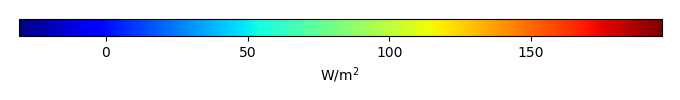MODEL MEANBIAS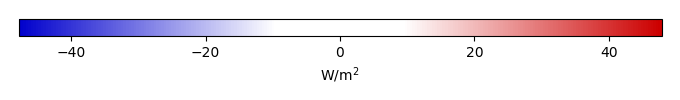BIAS SCORERMSE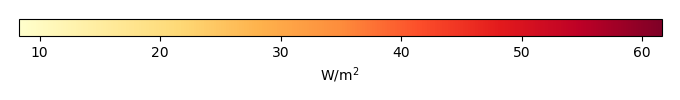RMSE SCOREBENCHMARK INTERANNUAL VARIABILITY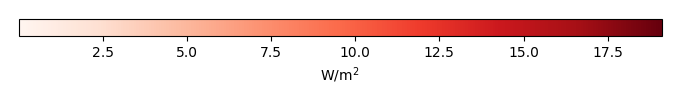MODEL INTERANNUAL VARIABILITYINTERANNUAL VARIABILITY SCOREBENCHMARK MAX MONTHMODEL MAX MONTHDIFFERENCE IN MAX MONTH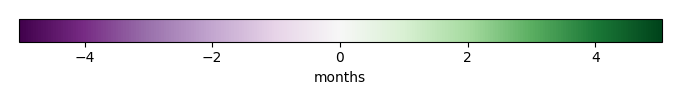SEASONAL CYCLE SCORESPATIAL TAYLOR DIAGRAMMODEL COLORS# Spatially integrated regional mean

MODEL COLORSREGIONAL MEANANNUAL CYCLEMONTHLY ANOMALYANNUAL CYCLE# All Models

BenchmarkCLM4CLM4.5CLM5# Data Information

creation_date: Thu May 8 22:58:12 PDT 2014

source_file: This product is generated from monthly 1 degree CERES EBAF Radiation observations